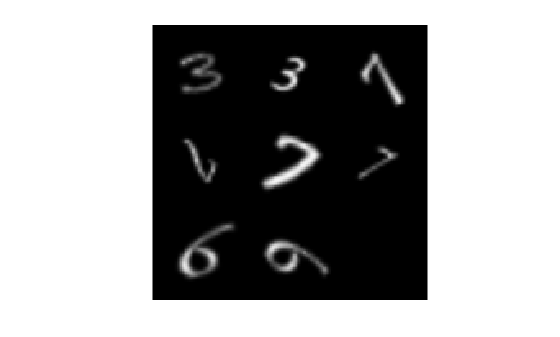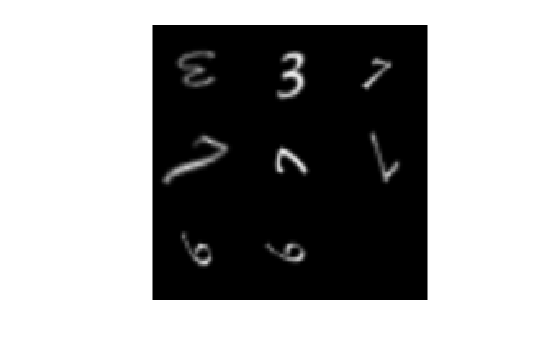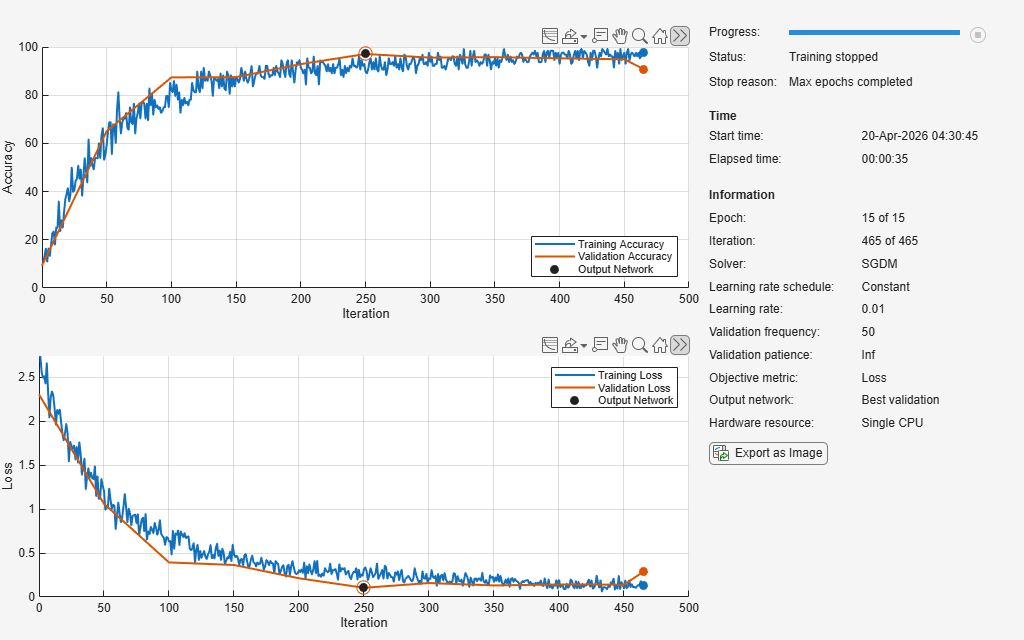# imageDataAugmenter

Configure image data augmentation

## Description

An image data augmenter configures a set of preprocessing options for image augmentation, such as resizing, rotation, and reflection.

The `imageDataAugmenter` is used by an `augmentedImageDatastore` to generate batches of augmented images. For more information, see Augment Images for Training with Random Geometric Transformations.

## Creation

### Syntax

``aug = imageDataAugmenter``
``aug = imageDataAugmenter(Name,Value)``

### Description

````aug = imageDataAugmenter` creates an `imageDataAugmenter` object with default property values consistent with the identity transformation.```

example

````aug = imageDataAugmenter(Name,Value)` configures a set of image augmentation options using name-value pairs to set properties. You can specify multiple name-value pairs. Enclose each property name in quotes.```

## Properties

expand all

Fill value used to define out-of-bounds points when resampling, specified as a numeric scalar or numeric vector.

• If the augmented images are single channel, then `FillValue` must be a scalar.

• If the augmented images are multichannel, then `FillValue` can be a scalar or a vector with length equal to the number of channels of the input image. For example, if the input image is an RGB image, `FillValue` can be a vector of length 3.

For grayscale and color images, the default fill value is `0`. For categorical images, the default fill value is an `'<undefined>'` label and the `trainnet` and `trainNetwork` functions ignore filled pixels when training.

Example: `128`

Random reflection in the left-right direction, specified as a logical scalar. When `RandXReflection` is `true` (`1`), each image is reflected horizontally with 50% probability. When `RandXReflection` is `false` (`0`), no images are reflected.

Random reflection in the top-bottom direction, specified as a logical scalar. When `RandYReflection` is `true` (`1`), each image is reflected vertically with 50% probability. When `RandYReflection` is `false` (`0`), no images are reflected.

Range of rotation, in degrees, applied to the input image, specified as one of the following.

• 2-element numeric vector. The second element must be larger than or equal to the first element. The rotation angle is picked randomly from a continuous uniform distribution within the specified interval.

• function handle. The function must accept no input arguments and return the rotation angle as a numeric scalar. Use a function handle to pick rotation angles from a disjoint interval or using a nonuniform probability distribution. For more information about function handles, see Create Function Handle.

By default, augmented images are not rotated.

Example: `[-45 45]`

Range of uniform (isotropic) scaling applied to the input image, specified as one of the following.

• 2-element numeric vector. The second element must be larger than or equal to the first element. The scale factor is picked randomly from a continuous uniform distribution within the specified interval.

• function handle. The function must accept no input arguments and return the scale factor as a numeric scalar. Use a function handle to pick scale factors from a disjoint interval or using a nonuniform probability distribution. For more information about function handles, see Create Function Handle.

By default, augmented images are not scaled.

Example: `[0.5 4]`

Range of horizontal scaling applied to the input image, specified as one of the following.

• 2-element numeric vector. The second element must be larger than or equal to the first element. The horizontal scale factor is picked randomly from a continuous uniform distribution within the specified interval.

• function handle. The function must accept no input arguments and return the horizontal scale factor as a numeric scalar. Use a function handle to pick horizontal scale factors from a disjoint interval or using a nonuniform probability distribution. For more information about function handles, see Create Function Handle.

By default, augmented images are not scaled in the horizontal direction.

Note

If you specify `RandScale`, then `imageDataAugmenter` ignores the value of `RandXScale` when scaling images.

Example: `[0.5 4]`

Range of vertical scaling applied to the input image, specified as one of the following.

• 2-element numeric vector. The second element must be larger than or equal to the first element. The vertical scale factor is picked randomly from a continuous uniform distribution within the specified interval.

• function handle. The function must accept no input arguments and return the vertical scale factor as a numeric scalar. Use a function handle to pick vertical scale factors from a disjoint interval or using a nonuniform probability distribution. For more information about function handles, see Create Function Handle.

By default, augmented images are not scaled in the vertical direction.

Note

If you specify `RandScale`, then `imageDataAugmenter` ignores the value of `RandYScale` when scaling images.

Example: `[0.5 4]`

Range of horizontal shear applied to the input image, specified as one of the following. Shear is measured as an angle in degrees, and is in the range (–90, 90).

• 2-element numeric vector. The second element must be larger than or equal to the first element. The horizontal shear angle is picked randomly from a continuous uniform distribution within the specified interval.

• function handle. The function must accept no input arguments and return the horizontal shear angle as a numeric scalar. Use a function handle to pick horizontal shear angles from a disjoint interval or using a nonuniform probability distribution. For more information about function handles, see Create Function Handle.

By default, augmented images are not sheared in the horizontal direction.

Example: `[0 45]`

Range of vertical shear applied to the input image, specified as one of the following. Shear is measured as an angle in degrees, and is in the range (–90, 90).

• 2-element numeric vector. The second element must be larger than or equal to the first element. The vertical shear angle is picked randomly from a continuous uniform distribution within the specified interval.

• function handle. The function must accept no input arguments and return the vertical shear angle as a numeric scalar. Use a function handle to pick vertical shear angles from a disjoint interval or using a nonuniform probability distribution. For more information about function handles, see Create Function Handle.

By default, augmented images are not sheared in the vertical direction.

Example: `[0 45]`

Range of horizontal translation applied to the input image, specified as one of the following. Translation distance is measured in pixels.

• 2-element numeric vector. The second element must be larger than or equal to the first element. The horizontal translation distance is picked randomly from a continuous uniform distribution within the specified interval.

• function handle. The function must accept no input arguments and return the horizontal translation distance as a numeric scalar. Use a function handle to pick horizontal translation distances from a disjoint interval or using a nonuniform probability distribution. For more information about function handles, see Create Function Handle.

By default, augmented images are not translated in the horizontal direction.

Example: `[-5 5]`

Range of vertical translation applied to the input image, specified as one of the following. Translation distance is measured in pixels.

• 2-element numeric vector. The second element must be larger than or equal to the first element. The vertical translation distance is picked randomly from a continuous uniform distribution within the specified interval.

• function handle. The function must accept no input arguments and return the vertical translation distance as a numeric scalar. Use a function handle to pick vertical translation distances from a disjoint interval or using a nonuniform probability distribution. For more information about function handles, see Create Function Handle.

By default, augmented images are not translated in the vertical direction.

Example: `[-5 5]`

## Object Functions

 `augment` Apply identical random transformations to multiple images

## Examples

collapse all

Create an image data augmenter that preprocesses images before training. This augmenter rotates images by random angles in the range [0, 360] degrees and resizes images by random scale factors in the range [0.5, 1].

```augmenter = imageDataAugmenter( ... 'RandRotation',[0 360], ... 'RandScale',[0.5 1])```
```augmenter = imageDataAugmenter with properties: FillValue: 0 RandXReflection: 0 RandYReflection: 0 RandRotation: [0 360] RandScale: [0.5000 1] RandXScale: [1 1] RandYScale: [1 1] RandXShear: [0 0] RandYShear: [0 0] RandXTranslation: [0 0] RandYTranslation: [0 0] ```

Create an augmented image datastore using the image data augmenter. The augmented image datastore also requires sample data, labels, and an output image size.

```[XTrain,YTrain] = digitTrain4DArrayData; imageSize = [56 56 1]; auimds = augmentedImageDatastore(imageSize,XTrain,YTrain,'DataAugmentation',augmenter)```
```auimds = augmentedImageDatastore with properties: NumObservations: 5000 MiniBatchSize: 128 DataAugmentation: [1x1 imageDataAugmenter] ColorPreprocessing: 'none' OutputSize: [56 56] OutputSizeMode: 'resize' DispatchInBackground: 0 ```

Preview the random transformations applied to the first eight images in the image datastore.

```minibatch = preview(auimds); imshow(imtile(minibatch.input));```Preview different random transformations applied to the same set of images.

```minibatch = preview(auimds); imshow(imtile(minibatch.input));```Train a convolutional neural network using augmented image data. Data augmentation helps prevent the network from overfitting and memorizing the exact details of the training images.

Load the sample data, which consists of synthetic images of handwritten digits.

`[XTrain,YTrain] = digitTrain4DArrayData;`

`digitTrain4DArrayData` loads the digit training set as 4-D array data. `XTrain` is a 28-by-28-by-1-by-5000 array, where:

• 28 is the height and width of the images.

• 1 is the number of channels.

• 5000 is the number of synthetic images of handwritten digits.

`YTrain` is a categorical vector containing the labels for each observation.

Set aside 1000 of the images for network validation.

```idx = randperm(size(XTrain,4),1000); XValidation = XTrain(:,:,:,idx); XTrain(:,:,:,idx) = []; YValidation = YTrain(idx); YTrain(idx) = [];```

Create an `imageDataAugmenter` object that specifies preprocessing options for image augmentation, such as resizing, rotation, translation, and reflection. Randomly translate the images up to three pixels horizontally and vertically, and rotate the images with an angle up to 20 degrees.

```imageAugmenter = imageDataAugmenter( ... 'RandRotation',[-20,20], ... 'RandXTranslation',[-3 3], ... 'RandYTranslation',[-3 3])```
```imageAugmenter = imageDataAugmenter with properties: FillValue: 0 RandXReflection: 0 RandYReflection: 0 RandRotation: [-20 20] RandScale: [1 1] RandXScale: [1 1] RandYScale: [1 1] RandXShear: [0 0] RandYShear: [0 0] RandXTranslation: [-3 3] RandYTranslation: [-3 3] ```

Create an `augmentedImageDatastore` object to use for network training and specify the image output size. During training, the datastore performs image augmentation and resizes the images. The datastore augments the images without saving any images to memory. `trainNetwork` updates the network parameters and then discards the augmented images.

```imageSize = [28 28 1]; augimds = augmentedImageDatastore(imageSize,XTrain,YTrain,'DataAugmentation',imageAugmenter);```

Specify the convolutional neural network architecture.

```layers = [ imageInputLayer(imageSize) convolution2dLayer(3,8,'Padding','same') batchNormalizationLayer reluLayer maxPooling2dLayer(2,'Stride',2) convolution2dLayer(3,16,'Padding','same') batchNormalizationLayer reluLayer maxPooling2dLayer(2,'Stride',2) convolution2dLayer(3,32,'Padding','same') batchNormalizationLayer reluLayer fullyConnectedLayer(10) softmaxLayer classificationLayer];```

Specify training options for stochastic gradient descent with momentum.

```opts = trainingOptions('sgdm', ... 'MaxEpochs',15, ... 'Shuffle','every-epoch', ... 'Plots','training-progress', ... 'Verbose',false, ... 'ValidationData',{XValidation,YValidation});```

Train the network. Because the validation images are not augmented, the validation accuracy is higher than the training accuracy.

`net = trainNetwork(augimds,layers,opts);`## Version History

Introduced in R2017b